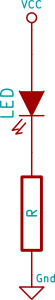Current Limiting Resistors

When using an LED the amount of current through the device controls how bright it is, but unless controlled the LED will draw more current than it is rated for, will damage itself, and probably the device it is pulling from (eg a microcontroller) as well.

To control how much current, and thus which brightness our LED is, we use a resistor.This circuit uses the fundamental Ohm's LawThe voltage across the resistor will be Vcc minus the Forward Voltage (Vf) of the LED, the amount of current to allow should be selected such that it is below the maximum the chip can supply, and below the maximum the LED can sustain.

From the PIC16F1544 data sheet we can see that a single pin can source or sink 25mA, and from the LED datasheet we can see its Steady Current is also 25mA. Also from the LED datasheet we get Vf of 2.2V, and our chosen Vcc is 5V. We will choose a moderate current below that limit, say 15 mA.

So if we rearrange the formula V=IR to find R then we getIn this case that is:Which gives us a suggested R of 187 ohm.

Resistors only come in certain values, so we will pick 220 ohm as a convenient approximation.

- Last change December 02, 2020, at 01:19 PM
- Registered in England and Wales 08777436